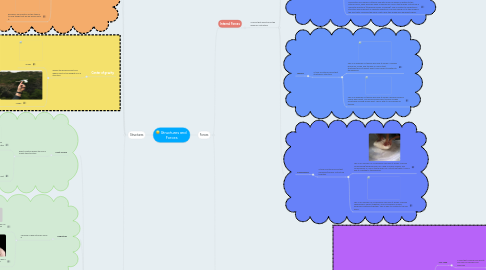# Structures and ForcesCynthia Xie
Get Started. It's FreeStructures and Forces## 1. Forces

### 1.1. Internal Forces

1.1.1. A force that affects only the inside of a structure

1.1.1.1. Torsion

1.1.1.1.1. A type of force that twists an object/structure

1.1.1.2. Shear

1.1.1.2.1. A type of internal force that pushes a structure in opposite directions

1.1.1.3. Tension

1.1.1.3.1. A type of internal force that stretches a structure

1.1.1.4. Compression

1.1.1.4.1. A type of internal force that squeezes/presses a structure together

### 1.2. External Forces

1.2.1. A force that only affects a structure on the outside

1.2.1.1.1. A external force that acts on a structure

## 2. Structures

### 2.1. Form

2.1.1. Basic shape of a structure

2.1.1.1. Shell Structure

2.1.1.1.1. A structure that is hollow on the inside

2.1.1.2. Combination Structure

2.1.1.2.1. A structure that contains a mixture of the other type of structures.

2.1.1.3. Frame Structure

2.1.1.3.1. A structure that is made of parts fastened together

2.1.1.4. Solid Strucure

2.1.1.4.1. A structure that is solid all the way through

### 2.2. Function

2.2.1. What the structure does/what is it used for

2.2.1.1. Example; The function of this chair is carrying the weight of people or objects that are going to be siting or placed on it.

2.2.1.2. Example; The function of this table is to hold things that will be placed onto it.

### 2.3. Center of gravity

2.3.1. Where the balance point and where most of the weight is on a structure

2.3.1.1. Image

2.3.1.2. Image

### 2.4. Effects On Structures

2.4.1. Point of App

2.4.1.1. Exact location where the force meets the structure

2.4.1.1.1. Example; This person is pushing this box exactly near the middle left of the box.

2.4.1.1.2. Example; This person is applying the force (poke) exactly on the top of the left arm.

2.4.2. Magnitude

2.4.2.1. Measure of how strong a force is

2.4.2.1.1. Example; The press is pressing down on the car with a high magnitude. (very strong)

2.4.2.1.2. Example; This person has a high magnitude in his punching.

2.4.3. Plane of Application

2.4.3.1. side of a structure affected by a force

2.4.3.1.1. This is an example of plane of application because it shows "panels" that show the sides of the person where a force can be applied. And the plane of application is a side which an applied force passes through.

2.4.3.1.2. This image is of a person, but it shows the sides of which a force can be applied to.

2.4.4. Direction

2.4.4.1. which way the force is applied to the structure

2.4.4.1.1. This image shows a person pushing a pole, and pulling the pole. This shows the direction of which the person is pushing/pulling; left and right.

2.4.4.1.2. This is an image of a person pushing a big weight in the direction up.

2.4.5. Speed of Applicaton

2.4.5.1. How fast the force is moving towards the structure.

2.4.5.1.1. Example; This boy is running really fast towards the wall

2.4.5.1.2. Example; The ball is moving really fast away from the person who threw it.

2.4.6. Angle of Application

2.4.6.1. The angle on the structure where the force is put

2.4.6.1.1. Example; This wreaking ball is going to hit this wall at a 90* angle

2.4.6.1.2. Example; This person is going to kick the ball at a 90* angle